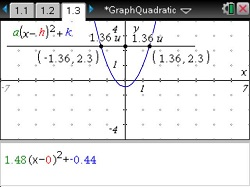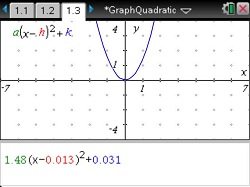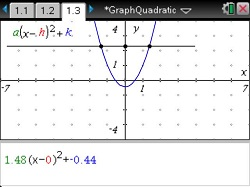# Activities

••• ##### Subject Area

• Math: Algebra I: Quadratic Functions

• ##### Author9-12

60 Minutes

• ##### Device
• TI-Nspire™ Navigator™
• TI-Nspire™
• TI-Nspire™ CAS
• ##### Software

TI-Nspire™
TI-Nspire™ CAS

3.0

• ##### Report an Issue#### Activity Overview

Students graph quadratic functions and study how the variables in the equations compare to the coordinates of the vertices and the axes of symmetry in the graphs.

#### Key Steps

•Students drag the expression x2 to the x axis to display the graph of the function y = x2.

•Students use the Parallel tool from the Construction menu to display a line parallel to the x-axis such that it intersects the parabola twice.

They then create the intersection points for where the parallel line intersects with the parabola and the y-axis.

•Next, they use the Length tool from the Measurement menu to measure the distances from each point on the parabola to the point on the y-axis.

Finally, they use the Coordinates and Equations tool from the Actions menu to display the coordinates of the points where the line intersects the parabola.